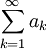# Theorem: The Integral Test

Previous: A Second Motivating Problem for The Integral Test

Next: An Integral Test Flowchart

Based on our two previous examples, you may have noticed that we were able to establish that the series converged or diverged because

• our functions were positive on some interval
• our functions were decreasing on some interval

In general, when applying the integral test, we do require these conditions, and also that our function is continuous. The conditions come out of the proof of the integral test.

## The Integral Test

The integral test is given by the following theorem.

Theorem: The Integral Test

Given the infinite seriesif we can find a function f(x) such that an=f(n) and that is continuous, positive, and decreasing on [1,∞), then the given series is convergent if and only ifconverges.

## Discussion

The integral test tells us that if the improper integralis convergent (that is, it is equal to a finite number), then the infinite series is convergent. If the improper integral is divergent (equals positive or negative infinity), then the infinite series is divergent. There are of course certain conditions needed to apply the integral test. Our function f must be positive, continuous, and decreasing, and must be related to our infinite series through the relation. However: when we encounter an infinite series that does not meet our criteria to apply the integral test, we can sometimes use some algebraic manipulation. We will explore this concept in a later example.

Previous: A Second Motivating Problem for The Integral Test

Next: An Integral Test Flowchart